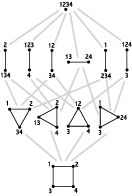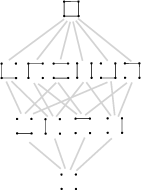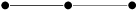Whitney NumbersI recently wrote a short C program to calculate the Whitney numbers of a graphical matroid very quickly.  Below is the source, the executable (for a Win98 DOS console, sorry!), and some sample graphs.  To run, simply double-click the executable, and give it the name of a graph file in the same directory as the executable when it asks for the filename.  Sorry about the lack of annotation and the somewhat sloppy coding: it was written in haste.  Feel free to clean it up and/or improve on my algorithms. Graph files are text files containing the adjacency matrix of a graph. Click here to download everything zipped up together (19K). Let me know if you see something interesting or make any significant improvements. So, what are Whitney numbers? The late Gian-Carlo Rota coined the term "Whitney numbers" to refer to the sizes of each of the rank-levels of a geometric lattice L, in honor of the combinatorialist and topologist Hassler Whitney, who more or less discovered/invented matroids.  That is, the nth Whitney number is the number of flats in L with rank n.  Don't know what a matroid or a geometric lattice is?  No sweat, I'll describe the concept for graphs, and you can go read more if you think it's interesting. If you have a graph G, and you identify two vertices that share an edge, you get a new graph, G0, as is pictured here:The result of a sequence of such identifications is a contraction.  Thus, the triangle pictured above is a contraction of the square.  We can picture all the contractions of a graph together, ordered by the order in which the identifications happened.  For example, the lattice of contractions of the square (also known as C4) looks like:Another way to view the lattice of contractions (the one I prefer, actually), is as follows.  A set S of vertices of a graph G is said to induce a subgraph H of G if H has S as its vertex set, and it has an edge between two elements of S precisely if G does.  A graph G is connected if there is a path from any vertex to any other vertex along edges of G.  A partition of a graph G is a splitting up of the vertices of G into sets, each of which induces a connected subgraph.  Order them by set inclusion.  The resulting lattice of partitions of  C4 looks like:See why they're really the same?  So, we say the element at the bottom has rank 1, the four at the next level have rank 2, the six at the next level have rank 3, and the one at the top has rank 4.  Note that the rank 2 elements (the atoms) are just the edges.  So, the lattice of contractions of C4 has Whitney numbers (1,4,6,1).  Let Tn be any tree on n vertices, that is, any graph on n vertices that has no cycles.  For example, T3 can be three vertices in a row:.  The following table lists their Whitney numbers (which do not depend on the choice of Tn): n W1 W2 W3 W4 W5 1 1 2 1 1 3 1 2 1 4 1 3 3 1 5 1 4 6 4 1 Recognize those numbers?  It's Pascal's triangle!  (Why?)  How about the complete graphs?  Kn is the graph on n vertices, where every two vertices are connected by an edge.  For example, K3 looks like a triangle, and K4 looks like a pyramid.  Their table of Whitney numbers is: n W1 W2 W3 W4 W5 1 1 2 1 1 3 1 3 1 4 1 7 6 1 5 1 15 25 10 1 Recognize those?  It's the Stirling numbers of the second kind.  Notice how, for the trees -- which are the (connected) graphs with the fewest edges -- the numbers increase and then decrease in each row, i.e., the binomial coefficients are unimodal; and how, for the complete graphs -- which are the graphs with the most edges -- the Whitney numbers are also unimodal.  This should happen for the graphs that fall in between the two, right?  This, in fact, is not known.  Rota conjectured in 1970 that the sequence of Whitney numbers for every graph is unimodal, but no one has been able to prove it or find a counterexample.  (In fact, he made the same conjecture about matroids, a more general structure than graphs.) The program I linked to above will calculate the Whitney numbers of any graph, since they are rather difficult to calculate by hand.  Try playing with it to get some intuition about the subject. For references on matroids (also known as combinatorial geometries, independence structures, and geometric lattices), see: Steve Pagano's matroid page, matroid references from Thomas Zaslavsky, or this page with lots of matroid theory and other combinatorics.

Last Edit: 9/7/2004